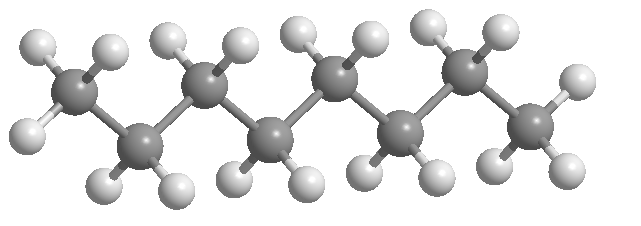Give the molecular formula, the condensed formula, and indicate the number of hydrogens each carbon has in the octane molecule.

SOLUTION:

An 8-carbon alkane has 18 hydrogens and its molecular formula is: C8H18 . The condensed formula is given by: CH2(CH2)nCH3 . The formula developed in which the carbons and hydrogens that the molecule has are indicated is:Octane molecular model: